# Mordell conjecture

Jump to: navigation, search

A conjecture on the finiteness of the set of rational points on an algebraic curve of genus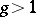. Advanced by L.J. Mordell  for the case when the ground fieldis the field of rational numbers. At present (1982) Mordell's conjecture is taken to be the assertion of the finiteness of the set of rational points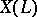of an irreducible algebraic curveof genusdefined over a fieldof finite type over the field of rational numbersin any finite extension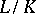. A reduction of Mordell's conjecture to the most difficult case whenis an algebraic number field has been obtained (see ). A number of special results related to Mordell's conjecture are known. Thus, it has been proved  thatis finite if the rank of the group of-automorphisms frominto an elliptic curveis larger than the rank of the group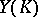. The finiteness of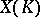has been established, , for the broad class of modular curves (cf. Modular curve) and their fields of definition. An estimate for the growth of the height,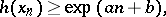of the rational pointshas been found, , showing that they are situated more "sparsely" than on the curves of genus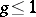. It has also been proved that Mordell's conjecture is a consequence of Shafarevich's conjecture on the finiteness of the number of algebraic curves having a given genus, a given field of definition (a finite extension of) and a given set of points of bad reduction (see , and also Siegel theorem on integer points).

The geometric analogue of Mordell's conjecture is the assertion of the finiteness of the number of sections of a bundle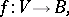whereis a non-singular projective surface,is a curve and the general fibre ofis an irreducible curve of genus. This assertion is true if the bundle is non-constant, that is, if it is not a direct product after a certain covering of the base, and if the characteristic of the ground fieldis equal to zero (see , ). For constant bundles it is possible only to assert the finiteness of the number of classes consisting of sections that are algebraically equivalent as curves on. If the characteristic ofis positive, the geometric analogue of Mordell's conjecture is false .